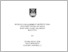# Lambda-max criteria weight determination in an adaptive neuro-fuzzy inference system / Rosma Mohd Dom, Daud Mohamad and Ajab Bai Akbarally

Mohd Dom, Rosma and Mohamad, Daud and Akbarally, Ajab Bai (2012) Lambda-max criteria weight determination in an adaptive neuro-fuzzy inference system / Rosma Mohd Dom, Daud Mohamad and Ajab Bai Akbarally. [Research Reports] (Unpublished)

## Abstract

Fuzzy inference is the process of formulating the mapping from a given input to an output using fuzzy logic. The primary mechanism for fuzzy inference engine involves a list of if- then statement called rules. A neuro-fuzzy system is a fuzzy system that uses learning algorithms derived from or inspired by neural network theory to determine its parameters (fuzzy sets and fuzzy rules) by processing data samples. In simple terms a neuro-fuzzy system is a combination of fuzzy logic and neural network in generating a model that produces required output (Oke S.A et a/., 2006). The existing method in determining the weights in the Adaptive Neuro-Fuzzy Inference System (ANFIS) is questionable since only simple multiplication (algebraic t-norm) is used. In fuzzy mathematics, criteria weights determination can be carried out using many available techniques such as the Technique for Order Preference by Similarity to Ideal Solution (TOPSIS), Analytic Hierarchy Process (AHP), Multi Attribute Utility Theory (MAUT), Multi Attribute Value Theory (MAVT) and the Fuzzy Preference Relation (FPR). There are three methods of calculating the fuzzy criteria weights using the Analytic Hierarchy Process (AHP) namely the Extend Analysis, Lambdamax and the Least Squares method. Each method has its own strengths and limitations. In this research we will use the Lamda-max method of the AHP to determine the criteria weights to replace the weights used in the existing ANFIS. Literatures have shown that the Extend Analysis method is not suitable when it involves extreme values (zero weights) and the Least Squares method requires an extensive amount of computing application. Thus the Lamda-max method is the most appropriate method to be used in this research. The first objective of this research is to improvise the existing ANFIS by applying the chosen criteria weight determination Lamda-max in developing the neuro-fuzzy system. The second objective is to assess the ability of the Modified ANFIS by comparing the performance of the Modified ANFIS with the Conventional ANFIS.In assessing the performance of Modified ANFIS we will use it to identify factors affecting the tensile strength of plastics. Currently experiments were carried out in the labs to help determine such contributing factors. The process can be very time consuming and costly. This research proposes a soft computing model specifically the Modified ANFIS model to help identify the contributing factors. The Modified ANFIS will be used to investigate the influence of fiber size, pH value, ash content, moisture content, pH value, density, melt flow rate and thermal transition on the tensile strength of plastics

Item Type: Research Reports
Creators:
CreatorsEmail / ID. Num
Mohd Dom, RosmaUNSPECIFIED
Akbarally, Ajab BaiUNSPECIFIED
Subjects: Q Science > QA Mathematics > Instruments and machines > Electronic Computers. Computer Science
Q Science > QA Mathematics > Fuzzy logic
Divisions: Universiti Teknologi MARA, Shah Alam > Research Management Centre (RMC)
Item ID: 21741
Uncontrolled Keywords: Lambda-max criteria weight; Neuro-fuzzy inference system
URI: http://ir.uitm.edu.my/id/eprint/21741Preview
Text
LP_ROSMA MOHD DOM RMI 12_5.pdf

## Fulltext

Fulltext is available at:
• UNSPECIFIED

## OthersView Item